# The high temperatures (in degrees Fahrenheit) of a randon sample of 10 small towns are: 96.7...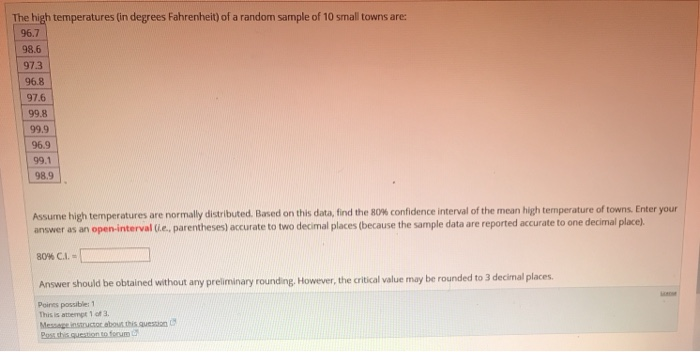The high temperatures (in degrees Fahrenheit) of a randon sample of 10 small towns are: 96.7 98.6 97.3 96.8 97.6 99.8 99.9 96.9 99.1 98.9 Assume high temperatures are normally distributed. Based on this data, find the 80% confidence interval of the mean high temperature of towns Enter your answer as an open-interval (ie, parentheses) acurate to two decimal places (because the sample data are reported accurate to one decimal place). 80% c.1. Answer should be obtained without any preliminary rounding, However, the critical value may be rounded to 3 decimal places Poires possible: 1 This is attempt 1 of 3. Poss shis question to fonum

Solution:- Given that values 96.7,98.6,97.3,96.8,97.6,99.8,99.9,96.9,99.1,98.9

x = 98.16 , S = 1.2456 , n = 10

df = n-1 = 10-1 = 9

t = 1.383

80% confidence interval of the mean high temperature : x +/- t*s/sqrt(n)
: 98.16 +/- 1.383*1.2456/sqrt(10)
: 97.615 , 98.705

##### Add Answer to: The high temperatures (in degrees Fahrenheit) of a randon sample of 10 small towns are: 96.7...
Similar Homework Help Questions
• ### The accompanying table lists body temperatures from 68 different randomly selected subjects measured at two different...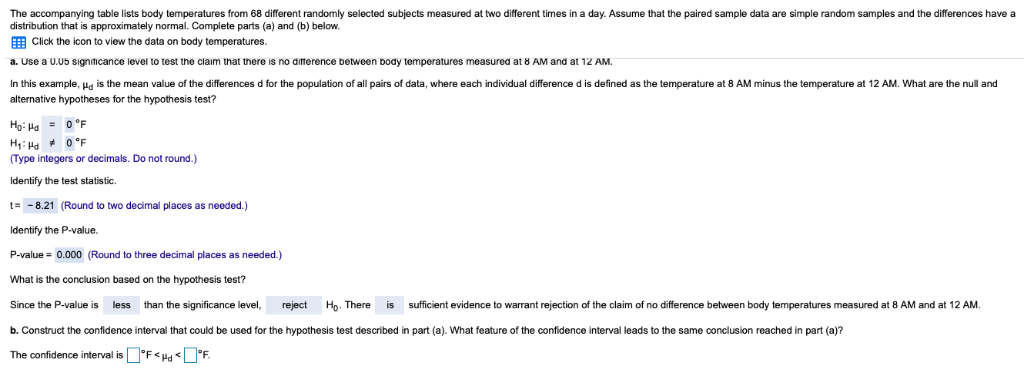The accompanying table lists body temperatures from 68 different randomly selected subjects measured at two different times in a day. Assume that the paired sample data are simple random samples and the differences have a distribution that is approximately normal. Complete parts (a) and (b) below. Click the icon to view the data on body temperatures. a. Use a 0.05 significance level to test the claim that there is no difference between body temperatures measured at 8 AM and at...

• ### The body temperatures in degrees Fahrenheit of a sample of adults in one small town are:...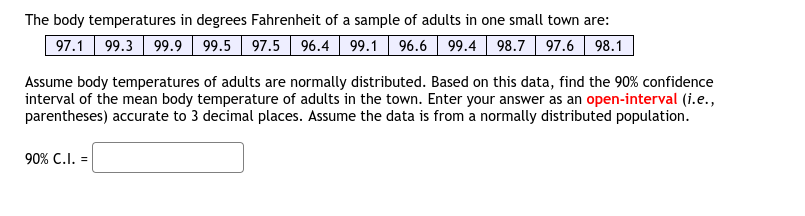The body temperatures in degrees Fahrenheit of a sample of adults in one small town are: 97.1 99.3 99.9 99.5 97.5 96.4 99.1 96.6 99.4 98.7 97.6 98.1 Assume body temperatures of adults are normally distributed. Based on this data, find the 90% confidence interval of the mean body temperature of adults in the town. Enter your answer as an open-interval (i.e., parentheses) accurate to 3 decimal places. Assume the data is from a normally distributed population. 90% C.1. =

• ### The body temperatures in degrees Fahrenheit of a sample of adults in one small town are:...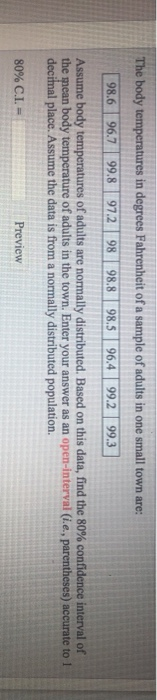The body temperatures in degrees Fahrenheit of a sample of adults in one small town are: 98.6 967 99.8 97.2 98 98.8 98 964 99.2 99.3 Assume body temperatures of adults are normally distributed. Based on this data, find the 80% confidence interval of the mean body temperature of adults in the town. Enter your answer as an open-interval (i.e., parentheses) accurate to 1 decimal place. Assume the data is from a normally distributed population. 80% CI,- Preview

• ### Refer to the data set of body temperatures in degrees Fahrenheit given in the accompanying table...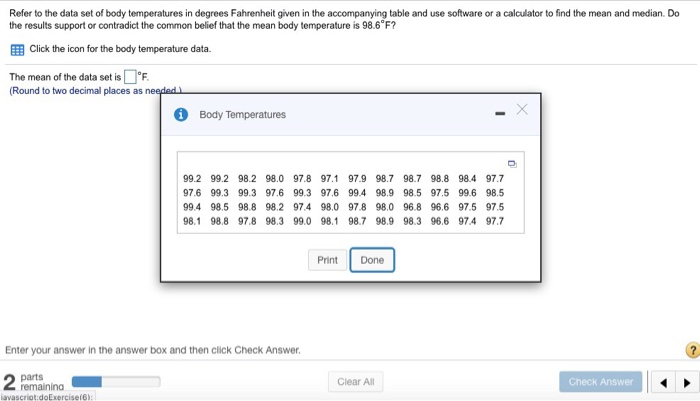Refer to the data set of body temperatures in degrees Fahrenheit given in the accompanying table and use software or a calculator to find the mean and median. Do the results support or contradict the common belief that the mean body temperature is 98.6 F? Click the icon for the body temperature data. The mean of the data set isF. Round to two decimall places as needed Body Temperatures 99.2 99.2 98.2 98.0 97.8 97.1 97.9 98.7 98.7 98.8 98.4...

• ### i Body Temperatures 97.4 96.9 98.2 98.8 99.4 98.5 96.5 98.598.2 96.6 98.8 99.5 98.199.1 98.397.2...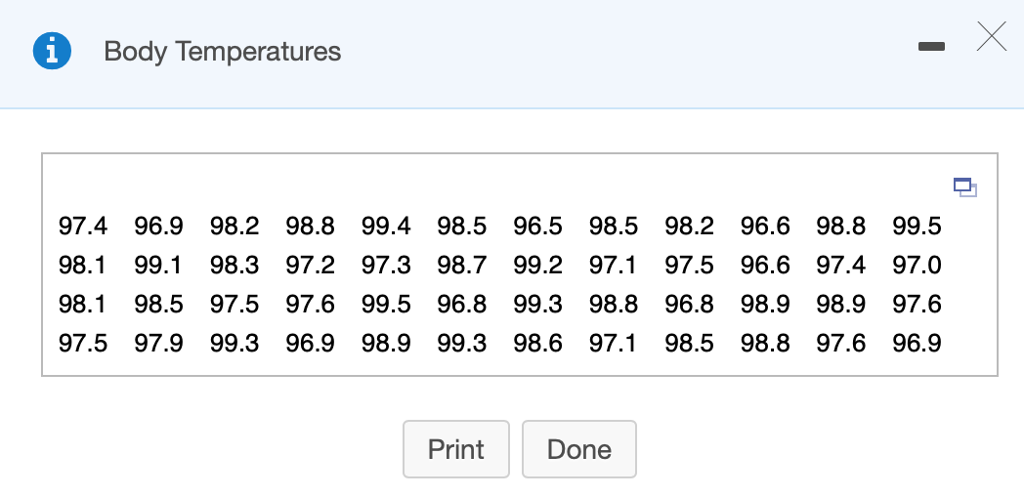i Body Temperatures 97.4 96.9 98.2 98.8 99.4 98.5 96.5 98.598.2 96.6 98.8 99.5 98.199.1 98.397.2 97.3 98.7 99.2 97.1 97.5 96.6 97.4 97.0 98.198.597.5 97.6 99.5 96.8 99.3 98.8 96.898.998.9 97.6 97.5 97.9 99.396.9 98.9 99.3 98.6 97.1 98.5 98.897.6 96.9 Print Done Refer to the data set of body temperatures in degrees Fahrenheit given in the accompanying table and use software or a calculator to find the mean and median. Do the results support or contradict the common...

• ### You want to obtain a sample to estimate a population mean. Based on previous evidence, you...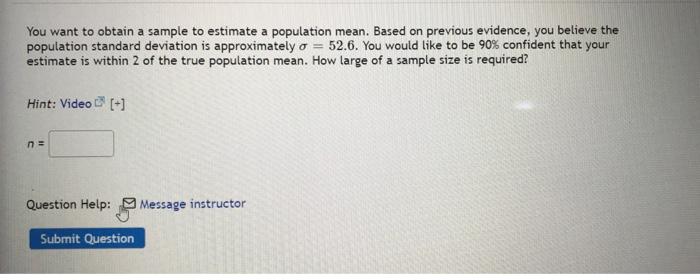You want to obtain a sample to estimate a population mean. Based on previous evidence, you believe the population standard deviation is approximately o = 52.6. You would like to be 90% confident that your estimate is within 2 of the true population mean. How large of a sample size is required? Hint: Video [+] n = Question Help: Message instructor Submit Question The body temperatures in degrees Fahrenheit of a sample of adults in one small town are: 96.3...

• ### You must estimate the mean temperature (in degrees Fahrenheit) with the following sample temperatures: 70.3 73.3...

You must estimate the mean temperature (in degrees Fahrenheit) with the following sample temperatures: 70.3 73.3 64.8 70 63.8 Find the 98% confidence interval. Enter your answer as an open-interval (i.e., parentheses) accurate to two decimal places (because the sample data are reported accurate to one decimal place). 98% C.I. = Answer should be obtained without any preliminary rounding.

• ### You must estimate the mean temperature (in degrees Fahrenheit) with the following sample temperatures: 73.5 64.1...

You must estimate the mean temperature (in degrees Fahrenheit) with the following sample temperatures: 73.5 64.1 45.3 53.9 58.7 Find the 99% confidence interval. Enter your answer as an open-interval (i.e., parentheses) accurate to two decimal places (because the sample data are reported accurate to one decimal place). 99% C.I. = Answer should be obtained without any preliminary rounding.

• ### You must estimate the mean temperature (in degrees Fahrenheit) with the following sample temperatures: 60.4 80.3...

You must estimate the mean temperature (in degrees Fahrenheit) with the following sample temperatures: 60.4 80.3 63.9 49.5 77.5 66.3 Find the 90% confidence interval. Enter your answer as an open-interval (i.e., parentheses) accurate to two decimal places (because the sample data are reported accurate to one decimal place). 90% C.I. = Answer should be obtained without any preliminary rounding.

• ### You must estimate the mean temperature (in degrees Fahrenheit) with the following sample temperatures: 5.2 18...

You must estimate the mean temperature (in degrees Fahrenheit) with the following sample temperatures: 5.2 18 17.2 20.7 26.9 33 Find the 98% confidence interval. Enter your answer as an open-interval (i.e., parentheses) accurate to two decimal places (because the sample data are reported accurate to one decimal place). 98% C.I. = Answer should be obtained without any preliminary rounding.

Free Homework App